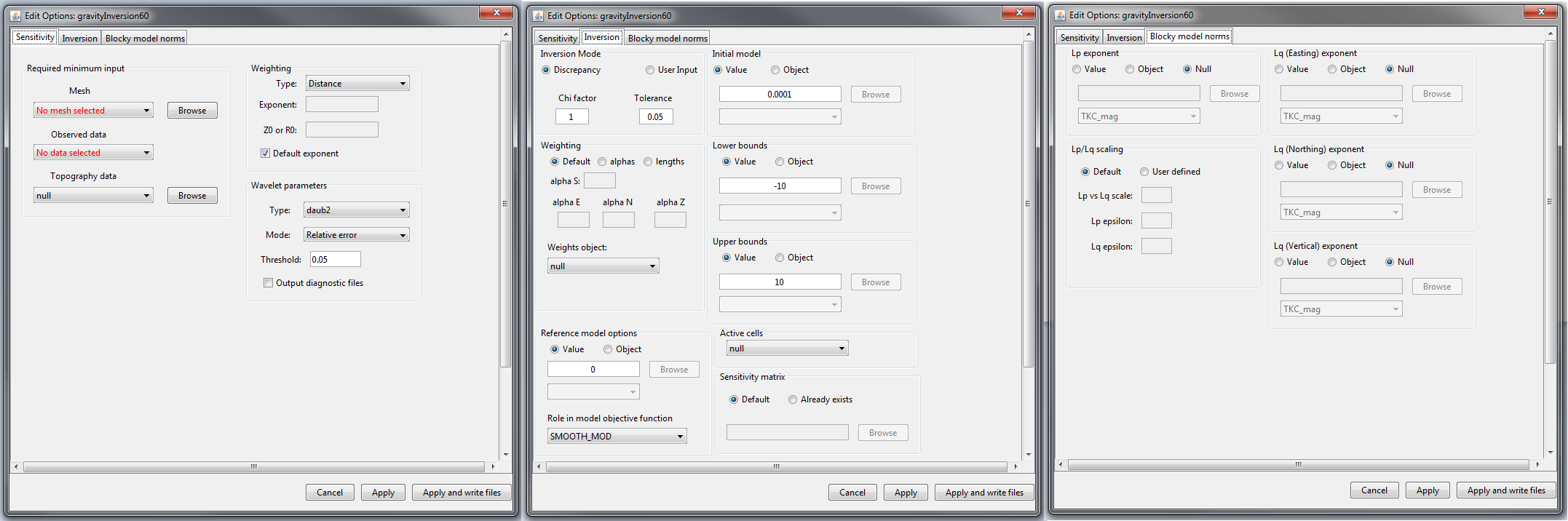# 4.8.2.1. Edit Options for Gravity Inversion Objects¶

## 4.8.2.1.1. Grav3D Inversion¶

This functionality is responsible for setting all inversion parameters pertaining to the 3D gravity inversion codes; see GRAV3D background theory. The edit options window is comprised of 3 tabs:Sensitivity (left), inversion (middle) and blocky model norms (right) tabs for GRAV inversion objects.

### 4.8.2.1.1.1. Units¶

Inputs:

• Observed data: gravity anomaly data given in units of milliGal (mGal)

• Reference/background density model: $$g/cm^3$$

Outputs:

• Recovered anomalous density model: $$g/cm^3$$

### 4.8.2.1.1.2. Sensitivity Tab¶

• Mesh: mesh for the recovered model

• Observed data: observed data including uncertainties

• Topography: a topography data object. Leave as null for flat topography at an elevation of 0 m.

• Weighting: set the type and parameters for sensitivity weighting. The parameters which define the sensitivity weighting are described in the Grav3D manual .

• Type: Depth or distance. The choice determines the expression used for the weighting

• Exponent: Given by $$\alpha$$ in the manual. This parameter has default = 2 to reflect the fact the fields fall of as $$1/r^2$$

• Z0 or R0: These constants are defined by equations in the manual. R0 and Z0 are small and generally chosen to be 1/4 the length of the smallest cell dimension

• Wavelet parameter: wavelet compression of the sensitivity matrix is used reduced the memory requirements for storing the sensitivity matrix and improve the speed of the inversion algorithm. The details of this are described in the Grav3D manual

• Type: sets the type of wavelet transform applied to the rows of the sensitivity matrix

• Mode:

• Relative error: the level of wavelet compression is specified by a relative threshold (default = 0.05)

• Threshold: the level of wavelet compression is specified an absolute threshold

• Threshold: user specified value based on choice in mode

### 4.8.2.1.1.3. Inversion Tab¶

• Inversion Mode: Sets the protocol for the trade-off parameter ($$\beta$$ )

• Discrepancy: sets the stopping criteria for the inversion using the discrepancy principle. Chi-factor determines the stopping criteria and tolerance sets how close to the ideal stopping criteria before the inversion is terminated.

• User Input: the user specifies the exact value for the trade-off parameter (beta )

• Weighting: Sets the weights for smallness and smoothness regularization in x, y and z; for relevant equations see manual .

• Default: Sets the values of alpha S, alpha X, alpha Y and alpha Z based on cell dimensions

• Alphas: Sets specific values for alpha S, alpha X, alpha Y and alpha Z

• Lengths: User sets values Len E, Len N and Len Z which define the values of alpha X, alpha Y and alpha Z relative to alpha S. These relationships are given by $$L_x = \sqrt{\frac{\alpha_x}{\alpha_s}}$$, $$L_y = \sqrt{\frac{\alpha_y}{\alpha_s}}$$ and $$L_z = \sqrt{\frac{\alpha_z}{\alpha_s}}$$.

• Weighting object: Specify additional cell weights. Use null if no additional model weights are supplied.

• Reference model options:

• Value: use a constant value to define the reference model

• Object: use a GIFmodel as the reference model

• Role in objective function: the user selects SMOOTH_MOD or SMOOTH_MOD_DIF. If SMOOTH_MOD is selected, the reference model is included only in the smallness term in the model objective function. If SMOOTH_MOD_DIF is selected, the reference model is included in the smallness and smoothness terms in the model objective function. Further explanation of this is found in fundamentals of inversion.

• Initial model:

• Value: use a constant value to define a homogeneous starting model

• Object: use a GIF model as the starting model

• Lower bounds:

• Value: set a constant value for the lower bounds for all cells

• Object: use a GIF model to supply cell-specific lower bounds

• Upper bounds:

• Value: set a constant value for the upper bounds for all cells

• Object: use a GIF model to supply cell-specific upper bounds

• Active cells: Specifies which cells lying below the surface topography are active during the inversion. All other cells remain fixed-valued (equal to starting model). Use null to set all cells lying below surface topography as active.

• Sensitivity matrix:

• Default: If this option is chosen, the code will generate the sensitivity matrix and save it to the working directory.

• Already exists: If the sensitivity matrix for your problem has already been created, this option allows the user to point to the sensitivity file and avoid recomputation

### 4.8.2.1.1.4. Blocky Model Norms Tab (ver. 5.1 and 6.0)¶

Sparse and blocky model norms are explained in fundamentals of inversion. Below are parameter descriptions for fields within edit options.

• Lp exponent:

• Value: set as a constant value $$p \in (0,2]$$

• Object: use a GIF model to supply cell-specific values for $$p$$

• Null: a default value of $$p=2$$ or $$q=2$$ is used

• Lq exponent (Easting, Northing or Vertical):

• Value: set as a constant value $$p \in (0,2]$$ or $$q \in (0,2]$$

• Object: use a GIF model to supply cell-specific values for $$p$$ or $$q$$

• Null: a default value of $$p=2$$ or $$q=2$$ is used

• Lp/Lq scaling: the nature of these parameters are discussed in fundamentals of inversion